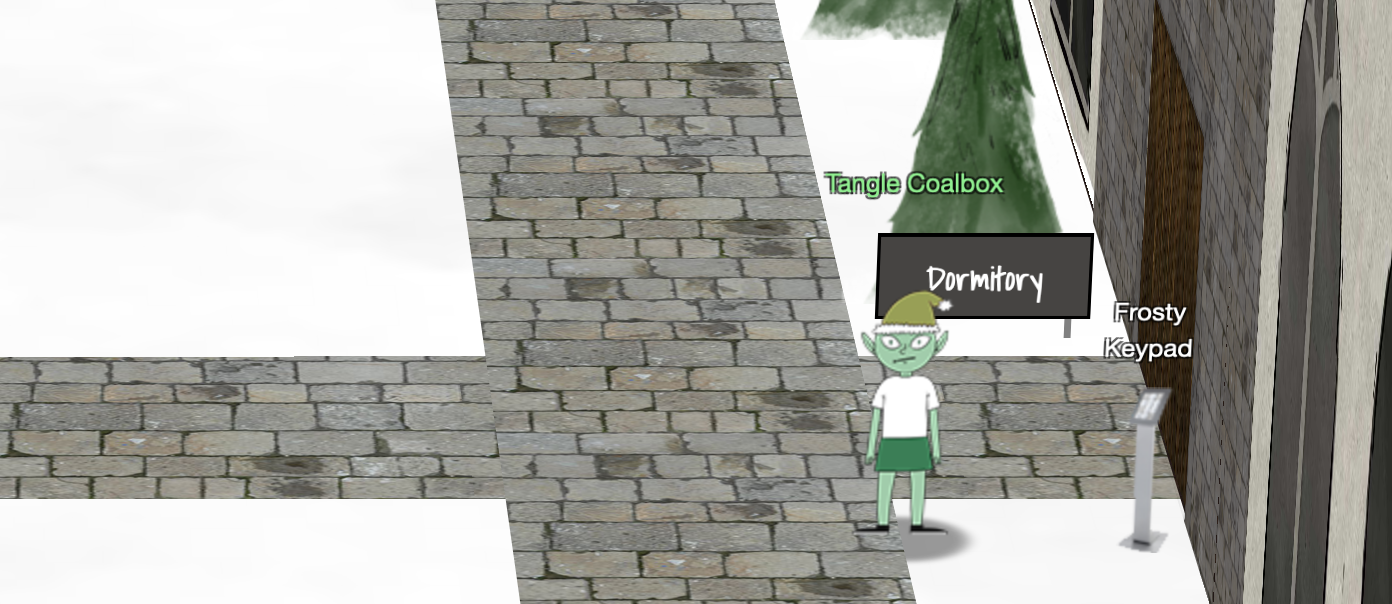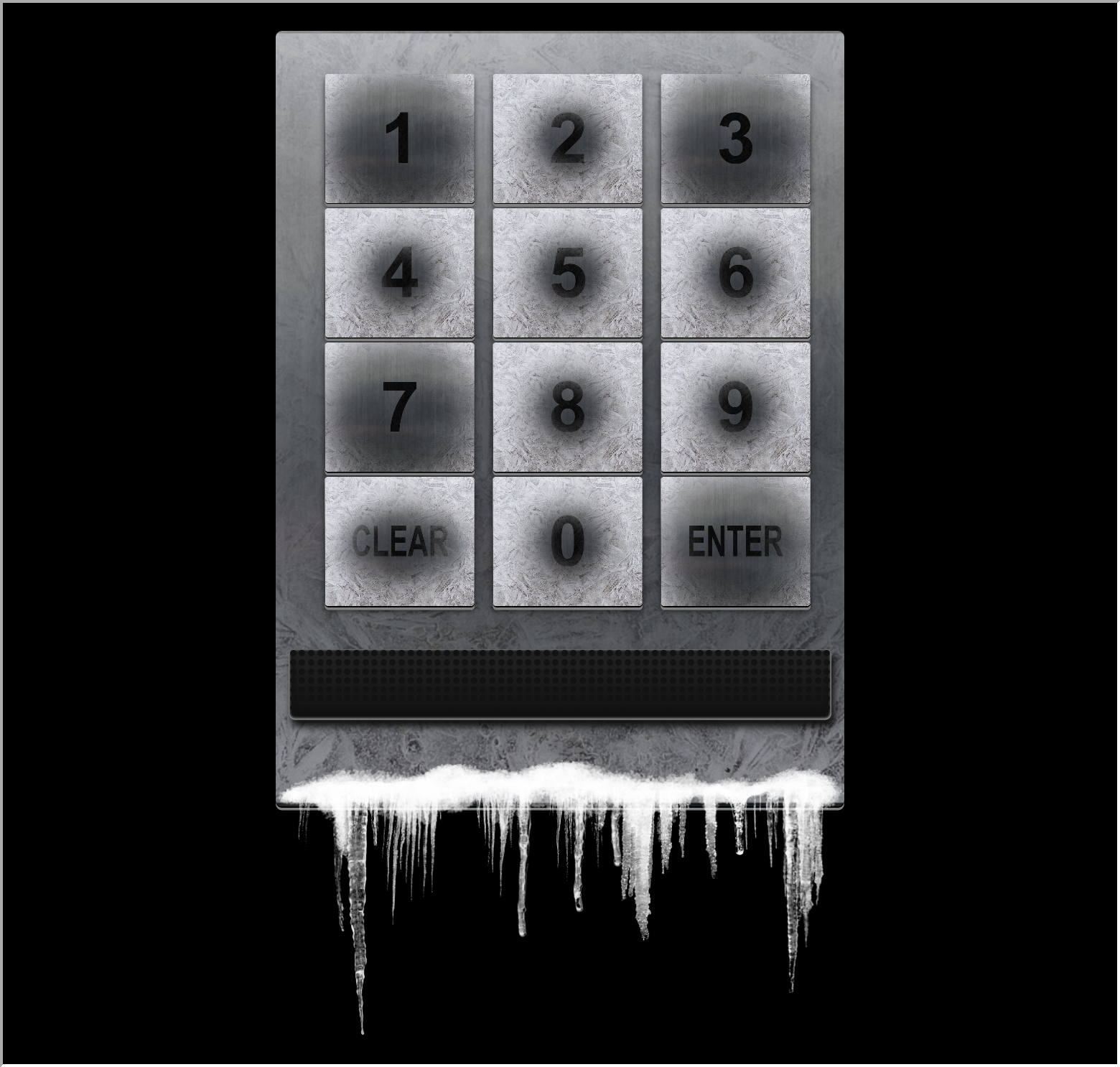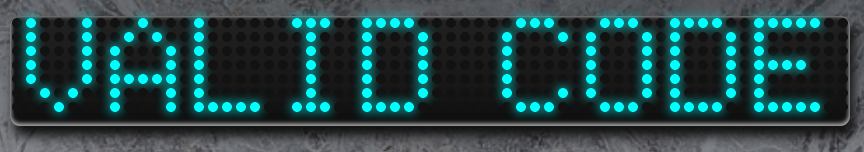🧝🏻‍♂️ Tangle Coalbox

Hey kid, it's me, Tangle Coalbox.
I'm sleuthing again, and I could use your help.
Ya see, this here number lock's been popped by someone.

I think I know who, but it'd sure be great if you could open this up for me.
I've got a few clues for you:

1. One digit is repeated once.
2. The code is a prime number.
3. You can probably tell by looking at the keypad which buttons are used.

One digit is repeated once, it's prime, and you can see which keys were used.

Get the correct key to open the door

### ⚡️ Solution¶

When You open the Keypad, You see:Given the hints, after looking at the keypad you can tell which buttons are used: `1, 3, 7`.

Also by the code must be 4 digits as One digit of the three is repeated once.

To we need get all possible combinations and check each code if it is a prim or not.

Hint

A prime number is a natural number greater than 1 that cannot be formed by multiplying two smaller natural numbers.1

We can use a small python code to help us:

```import itertools

# All numbers from the keypad and repeat the prime 3, 7
numbers = [1,3,7,3,7]

#Create all Possible Combinations
allnum =[]
for item in itertools.permutations(numbers,4):
num= ''.join([str(i) for i in item ])
num = int(num)
if num not in allnum:
allnum.append(num)
print("Possible Combinations", allnum)

# Check for Possible code by prime check
allprim = []
for num in allnum:
for i in range(2,num//2):
if (num % i) == 0:
break
else:
print(num, "is a prime number")
allprim.append(num)
print("Possible code:", allprim)
```

So we have 5 possible codes `1373`, `1733`, `3137`, `3371`, `7331`. Try each code.